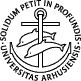UNIVERSITY OF AARHUS
BRICS INTERNATIONAL PHD SCHOOL

# Algorithms, Spring 2001 (Study group)Lecturer · Time and place · Course description · Literature · Handouts · Homework
Algorithms Fall 1999, 1998

## Lecturer

Gerth Stølting Brodal.

## Time and place

Wednesdays 14.15-16.00 in B4.18.

## Course description

The course is a graduate-level introduction to the design and analysis of algorithms. The aim of the course will be to explain basic methodological aspects such as amortized analysis of data structures, probabilistic analysis of algorithms or competitive analysis. To illustrate these, specific examples from important application areas - e.g. graph algorithms, string matching, or algebraic algorithms - will be discussed.
• Graph algorithms:
• Strongly connected componenets
• Max flow
• String matching: Knuth-Morris-Pratt algorithm
• Computational geometry: convex hulls, Voronoi diagrams
• Data structures:
• Hashing a' la Carter-Wegman
• Amortized analysis: binomial heaps, Fibonacci heaps
• Numerical algorithms:
• Finding best way to multiply matrices (dynamic programming)
• Solving systems of linear eq's
• FFT (and integeger multiplication via FFT)
• Randomization:
• Miller's primality test
• Computing maximal independent sets in parallel
• Skip lists, treaps
• Competitive analysis of paging heuristics
• Lower bounds:
• Sorting
Note: The syllabus given above is a bit ambitious; chances are that only a subset will be covered. The order given is not the one which will be followed. Furthermore, the content is subject to change according to contingencies.

## Literature

• Susanne Albers, Competitive Online Algorithms, BRICS LS-96-2, 1996.
• Sara Baase, Computer Algorithms: Introduction to Design and Analysis, 2nd Edition, Addison-Wesley, 1988,
• Timothy M. Chan, Optimal output-sensitive convex hull algorithms in two and three dimensions, Discrete and Computational Geometry, 16, pages 361-368, 1996.
• Thomas H. Cormen, Charles E. Leiserson, and Ronald L. Rivest, Introduction to Algorithms, MIT Press, 1990.
• Andrew V. Goldberg, Recent Developments in Maximum Flow Algorithms, 6th Skandinavian Workshop on Algorithm Theory (SWAT '98), LNCS 1432, pages 1-10, 1998.
• Dexter C. Kozen, The Design and Analysis of Algorithms, Springer-Verlag, 1992.
• Rajeev Motwani, Prabhakar Raghavan, Randomized Algorithms, Cambridge University Press, 1995.
• Sven Skyum, Introduction to lower bounds, 1997.
• Esko Ukkonen, On-Line Construction of Suffix Trees, Algorithmica, 14, pages 249-260, 1995.

## Homework

Page maintained by Gerth Stølting Brodal <gerth@cs.au.dk>.